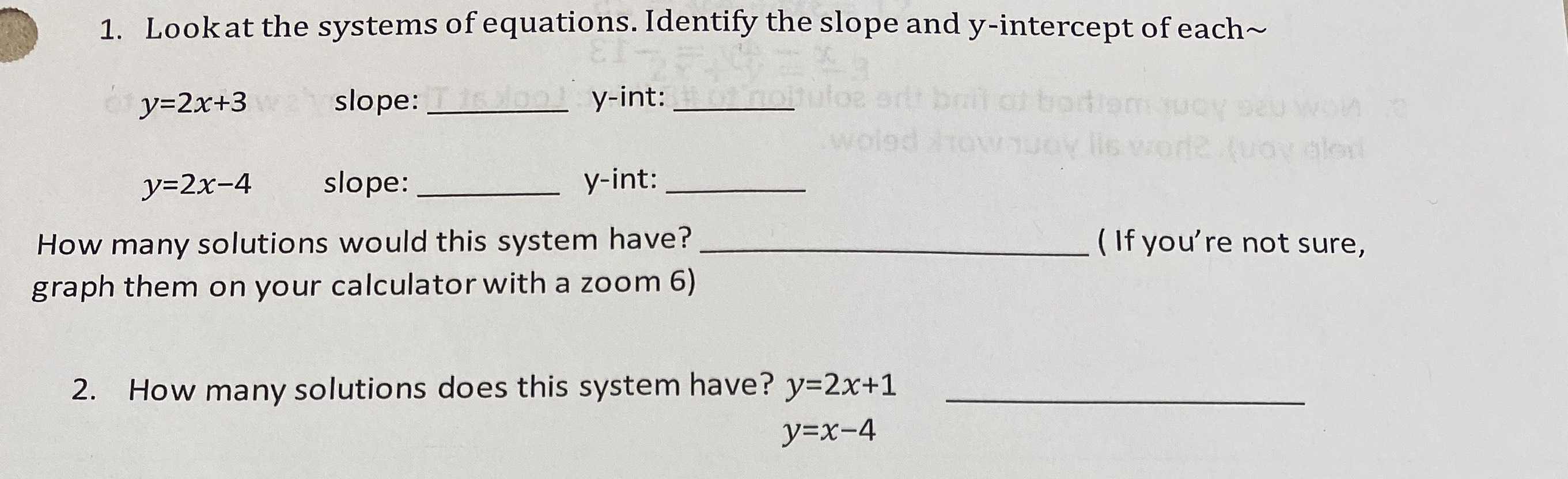### ¿Todavía tienes preguntas de matemáticas?

Pregunte a nuestros tutores expertos
Algebra
Pregunta1. Look at the systems of equations. Identify the slope and $$y$$ -intercept of each

$$y = 2 x + 3$$ slope: $$y$$ -int:

$$y = 2 x - 4$$ slope: How many solutions would this system have? graph them on your calculator with a zoom 6) 2. How many solutions does this system have? $$y = 2 x + 1$$

$$y = x - 4$$

1). For $$y= 2x+ 3$$, we have slope = 2 and y intercept is (0,3).
For $$y= 2x- 4$$, we have slope = 2 and y intercept is (0,-4).